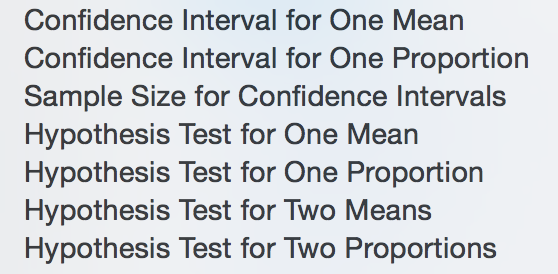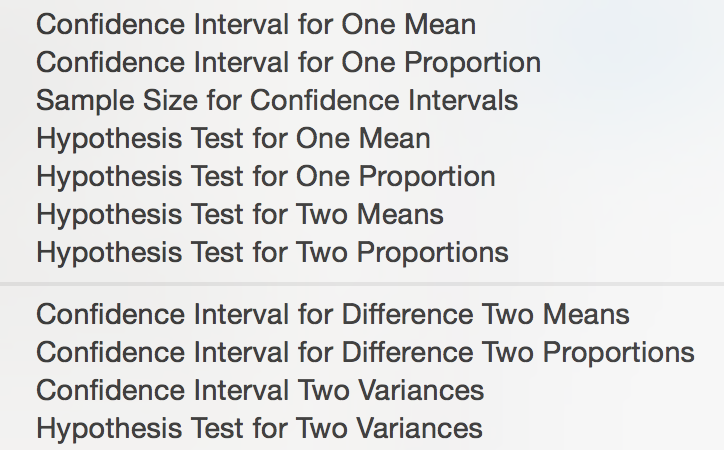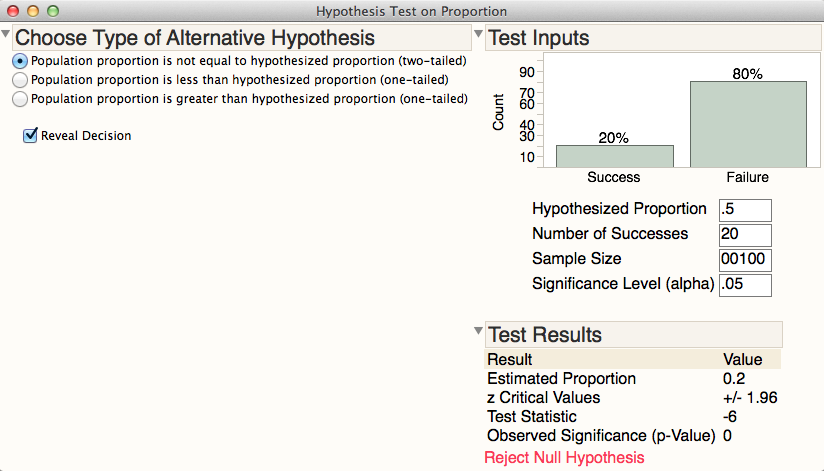This add-in provides calculators for confidence intervals, hypothesis tests, and sample size for means and proportions, using either summary statistics or raw data.  The add-in includes the following calculators, which are also available as scripts in JMP from Help > Sample Data, Calculators):Revision:  Sept 26, 2016

• Four additional calculators were added (these new calculators are not available in the Sample Data directory)
• Standard errors were added in some calculators
• Scripts were optimized for JMP 13 (but all scripts will run in JMP 12)

Revision: Sept 26, 2018

• Confidence Interval for One Proportion: Standard error is now correctly reported.The calculators are ideal for exploring how the width of confidence intervals change, how test results change, or how the calculated sample size changes as different input values are used:To perform ANOVA from summary statistics, see the ANOVA from Data Summary script developed by MarkBailey.